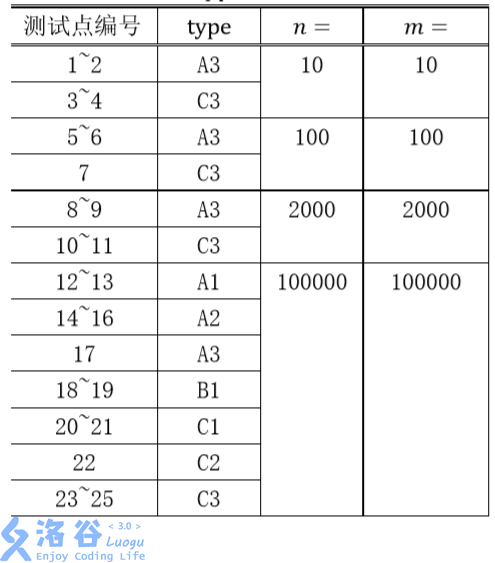P5024 保卫王国

• 951通过
• 12.1K提交
• 题目提供者 龟龟号打捞船 铲屎官
• 评测方式 云端评测
• 标签 倍增 动态规划,动规,dp 树链剖分,树剖 NOIp提高组 2018
• 难度 省选/NOI-
• 时空限制 2000ms / 512MB
• 提示：收藏到任务计划后，可在首页查看。

题目描述

Z 国有$n$座城市，$n - 1$条双向道路，每条双向道路连接两座城市，且任意两座城市 都能通过若干条道路相互到达。

Z 国的国防部长小 Z 要在城市中驻扎军队。驻扎军队需要满足如下几个条件：

• 一座城市可以驻扎一支军队，也可以不驻扎军队。
• 由道路直接连接的两座城市中至少要有一座城市驻扎军队。
• 在城市里驻扎军队会产生花费，在编号为i的城市中驻扎军队的花费是$p_i$。

小 Z 很快就规划出了一种驻扎军队的方案，使总花费最小。但是国王又给小 Z 提出 了$m$个要求，每个要求规定了其中两座城市是否驻扎军队。小 Z 需要针对每个要求逐一 给出回答。具体而言，如果国王提出的第$j$个要求能够满足上述驻扎条件（不需要考虑 第 $j$ 个要求之外的其它要求），则需要给出在此要求前提下驻扎军队的最小开销。如果 国王提出的第$j$个要求无法满足，则需要输出$-1 (1 ≤ j ≤ m)$。现在请你来帮助小 Z。

输入输出格式

输入格式：

第 $1$ 行包含两个正整数$n,m$和一个字符串$type$，分别表示城市数、要求数和数据类型。$type$是一个由大写字母 $A$，$B$ 或 $C$ 和一个数字 $1$，$2$，$3$ 组成的字符串。它可以帮助你获得部分分。你可能不需要用到这个参数。这个参数的含义在【数据规模与约定】中 有具体的描述。

第 $2$ 行$n$个整数$p_i$，表示编号$i$的城市中驻扎军队的花费。

接下来 $n - 1$ 行，每行两个正整数$u,v$，表示有一条$u$到$v$的双向道路。

接下来 $m$ 行，第$j$行四个整数$a,x,b,y(a ≠ b)$，表示第$j$个要求是在城市$a$驻扎$x$支军队， 在城市$b$驻扎$y$支军队。其中，$x$ 、 $y$ 的取值只有 $0$ 或 $1$：若 $x$ 为 $0$，表示城市 $a$ 不得驻 扎军队，若 $x$ 为 $1$，表示城市 $a$ 必须驻扎军队；若 $y$为 $0$，表示城市 $b$不得驻扎军队， 若 $y$为 $1$，表示城市 $b$ 必须驻扎军队。

输入文件中每一行相邻的两个数据之间均用一个空格分隔。

输出格式：

输出共 $m$ 行，每行包含 $1$ 个整数，第$j$行表示在满足国王第$j$个要求时的最小开销， 如果无法满足国王的第$j$个要求，则该行输出 $-1$。

输入输出样例

输入样例#1： 复制
5 3 C3
2 4 1 3 9
1 5
5 2
5 3
3 4
1 0 3 0
2 1 3 1
1 0 5 0
输出样例#1： 复制
12
7
-1

说明

【样例解释】

对于第一个要求，在 $4$ 号和 $5$ 号城市驻扎军队时开销最小。

对于第二个要求，在 $1$ 号、$2$ 号、$3$ 号城市驻扎军队时开销最小。

第三个要求是无法满足的，因为在 $1$ 号、$5$ 号城市都不驻扎军队就意味着由道路直接连 接的两座城市中都没有驻扎军队。

【数据规模与约定】

对于 $100\%$的数据，$n,m ≤ 100000,1 ≤ p_i ≤ 100000$。数据类型的含义：

$A$：城市$i$与城市$i + 1$直接相连。
$B$：任意城市与城市 $1$ 的距离不超过 $100$（距离定义为最短路径上边的数量），即如果这 棵树以 $1$ 号城市为根，深度不超过 $100$。
$C$：在树的形态上无特殊约束。
$1$：询问时保证$a = 1,x = 1$，即要求在城市 $1$ 驻军。对b,y没有限制。
$2$：询问时保证$a,b$是相邻的（由一条道路直接连通）
$3$：在询问上无特殊约束。

提示
标程仅供做题后或实在无思路时参考。
请自觉、自律地使用该功能并请对自己的学习负责。
如果发现恶意抄袭标程，将按照I类违反进行处理。